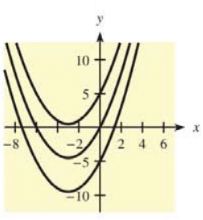Chapter 12.1, Problem 38E### Mathematical Applications for the ...

11th Edition
Ronald J. Harshbarger + 1 other
ISBN: 9781305108042

#### Solutions

Chapter
Section### Mathematical Applications for the ...

11th Edition
Ronald J. Harshbarger + 1 other
ISBN: 9781305108042
Textbook Problem

# In each of Problems 37-40, a family of functions is given and graphs of some members of the family are shown. Write the indefinite integral that gives the family. F ( x ) = x 2 2 + 3 x + CTo determine

To calculate: The indefinite integral which gives the family of the curves F(x)=x22+3x+C.Explanation

Given Information:

The provided equation is,

F(x)=x22+3x+C

And, the family of curves is,

Formula used:

According to the power rule of derivatives,

ddx(xn)=nxn1

Calculation:

Consider the provided function,

F(x)=x22+3x+C

Now, to obtain the indefinite integral that gives the above family of curves, first differentiate both sides with respect to x as,

ddx(F(x)

### Still sussing out bartleby?

Check out a sample textbook solution.

See a sample solution

#### The Solution to Your Study Problems

Bartleby provides explanations to thousands of textbook problems written by our experts, many with advanced degrees!

Get Started

#### Evaluate the indefinite integral. 2t2t+3dt

Single Variable Calculus: Early Transcendentals, Volume I

#### Convert from radians to degrees. 9. 512

Single Variable Calculus: Early Transcendentals

#### True or False: is a convergent series.

Study Guide for Stewart's Single Variable Calculus: Early Transcendentals, 8th